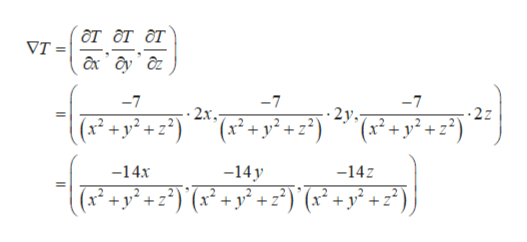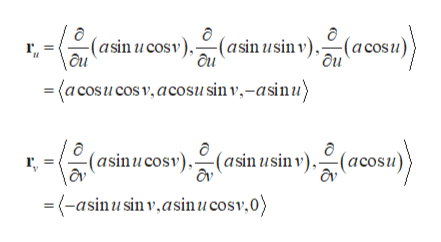The heat flow vector field for conducting objects is F=−k∇T​, where T(x,y,z) is the temperature in the object and k is a constant that depends on the material. Compute the outward flux of F across the given surface S for the given temperature distribution. Assume k=1. T(x,y,z,)=−7ln( x2 +y2 + z2)S is the sphere x2+y2+z2=a2

Question
The heat flow vector field for conducting objects is F=−k∇T​, where T(x,y,z) is the temperature in the object and k is a constant that depends on the material. Compute the outward flux of F across the given surface S for the given temperature distribution. Assume k=1.

T(x,y,z,)=−7ln( x2 +y2 + z2)
S is the sphere x2+y2+z2=a2
Step 1

Assume k = 1.

Then the vector field becomes, F = −∇T.

Find ∇T as follows.help_outlineImage Transcriptioncloseот от от УТ 3 ах ду д -7 -7 -7 2у. x* + y* +z*) ^ (x2? + y? +) 2х, 2z +y?+*) -14z -14х -14 у (x +y*+ z*)(x* +y? + z*) (* +y? +z°) } fullscreen
Step 2

The parametric description of the sphere of radius a, centered at the origin is given by,

Step 3

Take the partial derivative of the parameterization with...help_outlineImage Transcriptionclose(asin ucosv)(asin usin v), (acosu)} \au ' ôu (a cosucos v, acosusin v,-asinu) (asinucosv)(asin usin v),(acosu) 'av \av =(-asinusin v,asinucosv,0) fullscreen

Want to see the full answer?

See Solution

Want to see this answer and more?

Our solutions are written by experts, many with advanced degrees, and available 24/7

See Solution
Tagged in

Other